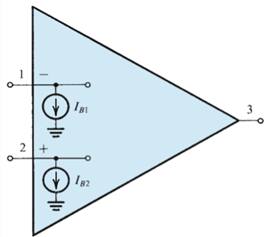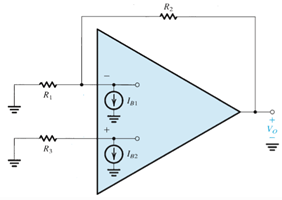Test: DC Imperfections in Operational Amplifiers

# Test: DC Imperfections in Operational Amplifiers

Test Description

## 10 Questions MCQ Test Analog Electronics | Test: DC Imperfections in Operational Amplifiers

Test: DC Imperfections in Operational Amplifiers for Electronics and Communication Engineering (ECE) 2022 is part of Analog Electronics preparation. The Test: DC Imperfections in Operational Amplifiers questions and answers have been prepared according to the Electronics and Communication Engineering (ECE) exam syllabus.The Test: DC Imperfections in Operational Amplifiers MCQs are made for Electronics and Communication Engineering (ECE) 2022 Exam. Find important definitions, questions, notes, meanings, examples, exercises, MCQs and online tests for Test: DC Imperfections in Operational Amplifiers below.
Solutions of Test: DC Imperfections in Operational Amplifiers questions in English are available as part of our Analog Electronics for Electronics and Communication Engineering (ECE) & Test: DC Imperfections in Operational Amplifiers solutions in Hindi for Analog Electronics course. Download more important topics, notes, lectures and mock test series for Electronics and Communication Engineering (ECE) Exam by signing up for free. Attempt Test: DC Imperfections in Operational Amplifiers | 10 questions in 10 minutes | Mock test for Electronics and Communication Engineering (ECE) preparation | Free important questions MCQ to study Analog Electronics for Electronics and Communication Engineering (ECE) Exam | Download free PDF with solutions
 1 Crore+ students have signed up on EduRev. Have you?
Test: DC Imperfections in Operational Amplifiers - Question 1

### Consider an inverting amplifier with a nominal gain of 1000 constructed from an op amp with an input offset voltage of 3 mV and with output saturation levels of ±10 V. What is (approximately) the peak sine-wave input signal that can be applied without output clipping?

Detailed Solution for Test: DC Imperfections in Operational Amplifiers - Question 1

The maximum that can be sent without clipping is 10V – 1000 X 3mV or 7V.

Test: DC Imperfections in Operational Amplifiers - Question 2

### (Q2 & Q.3) Consider an inverting amplifier with a nominal gain of 1000 constructed from an op amp with an input offset voltage of 3 mV and with output saturation levels of ±10 V. If the effect of VOs(input offset voltage) is nulled at room temperature (250C), how large an input can one now apply if: Q. The circuit is to operate at a constant temperature?

Detailed Solution for Test: DC Imperfections in Operational Amplifiers - Question 2

Explanation: Maximum signal that will not be clipped is 10mV because 10mV X 1000 = 10V.

Test: DC Imperfections in Operational Amplifiers - Question 3

### The circuit is to operate at a temperature in the range 0°C to 75°C and the temperature coefficient of VOS is 10 μV/°C?

Detailed Solution for Test: DC Imperfections in Operational Amplifiers - Question 3

Since the effect is nullified at 25oC, the peak that can be sent now is given by 10 – (75-25) X 0.1 mV.

Test: DC Imperfections in Operational Amplifiers - Question 4

One of the DC imperfections of the amplifiers are dc offset voltage which is

Detailed Solution for Test: DC Imperfections in Operational Amplifiers - Question 4

DC offset voltage is existence of output signal even when the differential signal is zero.

Test: DC Imperfections in Operational Amplifiers - Question 5

For the amplifier shown determine the value of the bias current (Ib) and input offset current (Io) respectively.Detailed Solution for Test: DC Imperfections in Operational Amplifiers - Question 5

Standard mathematical expressions are used with the given variables.

Test: DC Imperfections in Operational Amplifiers - Question 6

Consider the circuit shown below which reduces the impact of the input bias current. If IB1 = IB2 = Input bias current, then determine the value of R3 so that the output voltage (v0) is not impacted by the input bias current.Detailed Solution for Test: DC Imperfections in Operational Amplifiers - Question 6

This will be possible when R3 has the same value as the net effect of R1 and R2.

Test: DC Imperfections in Operational Amplifiers - Question 7

Consider an inverting amplifier circuit designed using an op amp and two resistors, R1 = 10 kΩ and R2 = 1 MΩ. If the op amp is specified to have an input bias current of 100 nA and an input offset current of 10 nA, find the output dc offset voltage resulting.

Detailed Solution for Test: DC Imperfections in Operational Amplifiers - Question 7

Use the mathematical definition of bias current and offset current.

Test: DC Imperfections in Operational Amplifiers - Question 8

(Q.8-Q.10) Consider a Miller integrator with a time constant of 1ms and an input resistance of 10 kΩ. Let the op amp have VOS (offset voltage) = 2 mV and output saturation voltages of ±12 V.​

Q. Assuming that when the power supply is turned on the capacitor voltage is zero, how long does it take for the amplifier to saturate?

Detailed Solution for Test: DC Imperfections in Operational Amplifiers - Question 8

Use vO = VOS
(VOS/CR)t.

Test: DC Imperfections in Operational Amplifiers - Question 9

Select the largest possible value for a feedback resistor RF so that at least ±10 V of output signal swing remains available.

Detailed Solution for Test: DC Imperfections in Operational Amplifiers - Question 9

Use vO = VOS
(VOS/CR)t.

Test: DC Imperfections in Operational Amplifiers - Question 10

What is the corner frequency of the resulting STC network?

Detailed Solution for Test: DC Imperfections in Operational Amplifiers - Question 10

The required answer is given by 1/6 Hz.

## Analog Electronics

11 videos|57 docs|97 tests
 Use Code STAYHOME200 and get INR 200 additional OFF Use Coupon Code
Information about Test: DC Imperfections in Operational Amplifiers Page
In this test you can find the Exam questions for Test: DC Imperfections in Operational Amplifiers solved & explained in the simplest way possible. Besides giving Questions and answers for Test: DC Imperfections in Operational Amplifiers, EduRev gives you an ample number of Online tests for practice

## Analog Electronics

11 videos|57 docs|97 tests